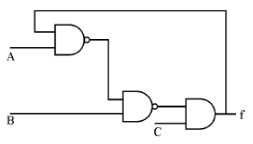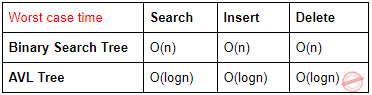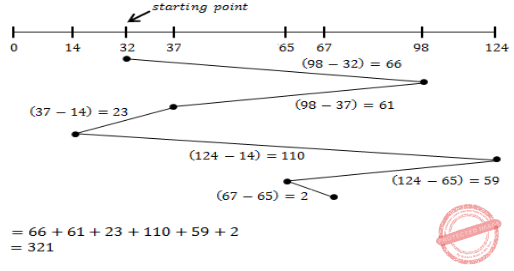## UGC NET CS 2012 Dec-Paper-2

 Question 1
Consider the circuit shown below. In a certain steady state, Y is at logical ‘1’. What are possible values of A, B, C ?A A = 0, B = 0, C = 1 B A = 0, B = C = 1 C A = 1, B = C = 0 D A = B = 1, C = 1 E A and D correct
Digital-Logic-Design       Logic-Gates
Question 1 Explanation:
The output f of the circuit is given as feedback input which should always be 1.
From the given circuit
f= (A+B')C (Assuming that feedback input is 1)
So, the output remains 1 iff C=1 and (A+B')=1.
A=1, B=0, C=1
A=1, B=1, C=1
A=0, B=0, C=1
Hence option A and D are correct.
 Question 2
The worst case time complexity of AVL tree is better in comparison to binary search tree for
 A Search and Insert Operations B Search and Delete Operations C Insert and Delete Operations D Search, Insert and Delete Operations
Data-Structures       Binary-Trees
Question 2 Explanation:
AVL tree is a self balancing tree with height difference at most 1. A balance factor in AVL tree is used to check what rotation to make.Question 3
The GSM network is divided into the following three major systems :
 A SS, BSS, OSS B BSS, BSC, MSC C CELL, BSC, OSS D SS, CELL, MSC
Data-Communication       Cellular-Networks
Question 3 Explanation:
GSM Architecture:Mobile Station (MS)
Mobile Equipment (ME)
Subscriber Identity Module (SIM)
Base Station Subsystem (BSS)
Base Transceiver Station (BTS)
Base Station Controller (BSC)
Network Switching Subsystem (NSS)
Mobile Switching Center (MSC)
Home Location Register (HLR)
Visitor Location Register (VLR)
Authentication Center (AUC)
Equipment Identity Register (EIR)
 Question 4
The power set of the set {ɸ} is
 A {ɸ} B {ɸ, {ɸ}} C {0} D {0, ɸ, {ɸ}}
Engineering-Mathematics       Set-Theory
Question 4 Explanation:
The given set is not a null set don't get confused in this.
A null set is a set which have no element in it and can be represented as either { } or ɸ.
And the given set {ɸ} represents a set having ɸ as its element. It is not a null set because given set is having ɸ inside it.
As we know power set is a set of all subsets of a given set. For given set it's power set is {ɸ, {ɸ}}
 Question 5
If the disk head is located initially at 32, find the number of disk moves required with FCFS if the disk queue of I/O blocks requests are 98, 37, 14,124, 65, 67.
 A 239 B 310 C 321 D 325
Operating-Systems       Disk-scheduling
Question 5 Explanation:Question 6
Component level design is concerned with
 A Flow oriented analysis B Class based analysis C Both of the above D None of the above
Question 6 Explanation:
Explanation:Question 7
The ‘C’ language is
 A Context free language B Context sensitive language C Regular language D None of the above
Theory-of-Computation       Languages-and-Grammars
Question 7 Explanation:
C and C++ are context sensitive languages.
 Question 8
The Mobile Application Protocol (MAP) typically runs on top of which protocol ?
 A SNMP (Simple Network Management Protocol) B SMTP (Simple Mail Transfer Protocol) C SS7 (Signalling System 7) D HTTP (Hypertext Transfer Protocol)
Computer-Networks       Application-Layer-Protocol
Question 8 Explanation:
→ The Mobile Application Protocol (MAP) is an SS7 protocol that provides an application layer for the various nodes in GSM and UMTS mobile core networks and GPRS core networks to communicate with each other in order to provide services to users.
→ The Mobile Application Protocol is the application-layer protocol used to access the Home Location Register, Visitor Location Register, Mobile Switching Center, Equipment Identity Register, Authentication Centre, Short message service center and Serving GPRS Support Node (SGSN).
 Question 9
If a packet arrive with an M-bit value is ‘1’ and a fragmentation offset value ‘0’, then it is ______ fragment.
 A First B Middle C Last D All of the above
Computer-Networks       IPv4-an-Fragmentation
Question 9 Explanation:
M bit is 1: It is either the first fragment or a middle one because of the offset value is 0, it is the first fragment.
M bit is 0: It means there are no more fragments. The fragment is the last one.
 Question 10
The number of bit strings of length eight that will either start with a 1 bit or end with two bits 00 shall be
 A 32 B 64 C 128 D 160
Engineering-Mathematics       Combinatorics
Question 10 Explanation:
→ Number of bit strings of length 8 that start with 1: 27 = 128.
→ Number of bit strings of length 8 that end with 00: 26 = 64.
→ Number of bit strings of length 8 that start with 1 and end with 00: 25 = 32.
→ Applying the subtraction rule, the number is 128+64−32 = 160
 Question 11
In compiler design ‘reducing the strength’ refers to
 A reducing the range of values of input variables. B code optimization using cheaper machine instructions. C reducing efficiency of program. D None of the above
Compiler-Design       Compilers
Question 11 Explanation:
In compiler design ‘reducing the strength’ refers to code optimization using cheaper machine instructions.
Example:
Dividing by 2→ Use right shift by 2.
Multiplication by 2→ Use left shift by 2.
 Question 12
In which addressing mode, the effective address of the operand is generated by adding a constant value to the contents of register ?
 A Absolute B Indirect C Immediate D Index
Question 12 Explanation:
1. An absolute address is represented by the contents of a register. This addressing mode is absolute in the sense that it is not specified relative to the current instruction address.
2. Indirect addressing is a scheme in which the address specifies which memory word or register contains not the operand but the address of the operand.
Immediate Operand:
The simplest way for an instruction to specify an operand is for the address part of the instruction actually to contain the operand itself rather than an address or other information describing where the operand is. Such an operand is called an immediate operand because it is automatically fetched from memory at the same time the instruction itself is fetched. It is immediately available for use.
Index mode:
The address of the operand is obtained by adding to the contents of the general register (called index register) a constant value. The number of the index register and the constant value are included in the instruction code
There are 12 questions to complete.

Register Now